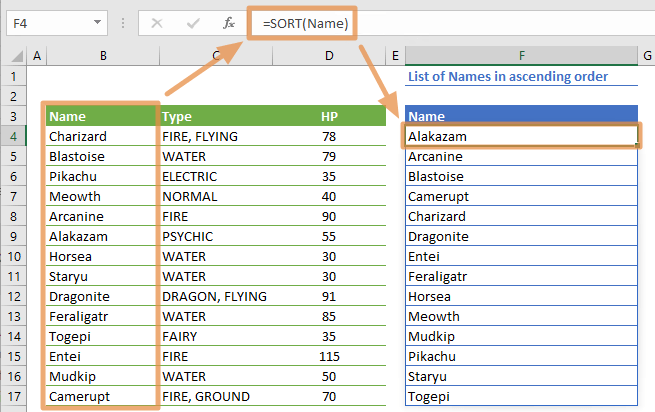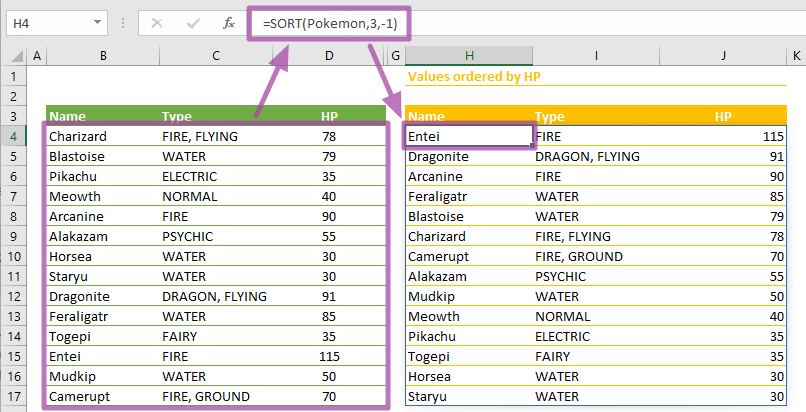The Excel SORT function is a lookup and reference formula that sorts the values in a range or array. The SORT function is one of the newest Dynamic Array functions which are release with Excel 2019. In this guide, we’re going to show you how to use the SORT function and also go over some tips and error handling methods.

The Dynamic Array functions can populate an array of values in a cell range based on another formula. This behavior is called spilling and can help overcome the limitations of array formulas.

# Supported versions

• At the time of writing this article, Microsoft announced that this formula is currently only available to a number of select insider users. When it's ready, the feature is planned for release for Office 365 users.

# Syntax

=SORT(array,[sort_index],[sort_order],[by_col])

# Arguments

 array The range or array that you want to sort. [sort_index] Optional. A numeric value indicating whether the list is to be sorted by row or column. The default value is 1. [sort_order] Optional. A numeric value indicating the sorting order. Ascending: 1 (default) Descending: -1 [by_col] Optional. A Boolean value that specifies the sorting direction. By row = FALSE (default) By column = TRUE.

Examples

Note that we're using named ranges in our sample formulas to make them easier to read. This is not required.

## Basic usage

=SORT(Name)
formula is a simple use case with the Excel SORT function. Enter the range of values for array argument and press Enter. Excel will populate the sorted list automatically. If you use the range without any other parameters, the rows will be sorted in an ascending order by default. You do not need to select the range or specify how many items are to be listed. If there is enough space (empty cells) underneath the formula, the formula will work as intended.## Sort by any column in the desired order

=SORT(Pokemon,3,-1)
formula is a demonstration for using the [sort_index] and [sort_order] parameters. You can sort a range with multiple columns by a specific column in the desired order. For example, setting [sort_index] 3 and [sort_order] -1 means that the range will be sorted by its 3rd column in the descending order. If you apply these parameters to the sample formula here, you will get a table sorted by column HP from high to low.## Sort by multiple column

=SORT(Pokemon,{2,1},{-1,1})
formula shows how the SORT formula can sort a range of values based on multiple columns. To perform sorting with more than one column, you need to enter those for [sort_index]. You can use arrays for this step. If we set {2,1} array to [sort_index], we can get a sorted listed ordered by the 2nd column and the 1st column respectively. This means that you can specify the ordering priority between columns by entering them in that order.

You can use different ordering types for different columns by entering another array into the [sort_order] argument. Once again, the order of the arguments are important. The first value in the array determines the first column of the array in the [sort_index]. As a result, entering {-1,1} to the [sort_order] means sorting the 2nd column in descending order and the 1st column in ascending order.## Sort top 3 column

=INDEX(SORT(Pokemon,3,-1),SEQUENCE(3),1)
formula uses the INDEX and the SEQUENCE functions to help SORT function list only the top 3 values of the 1st column, which is sorted by the 3rd column. In this example, the SORT function part is the same as the formula in the Sort by any column in desired order section. In some cases, you may not want to populate the full list. For this, you need to enter an array that only includes the row (or column according to your order direction) indexes for the INDEX function. For the top 3 rows, the array should be {1;2;3}. You can use the SEQUENCE function to create this array quickly. You do not need to enter 10 numbers to get a top ten list, just enter the number you need into the SEQUENCE formula.# Tips

• Currently, this formula is only available on Office 365 which comes with a subscription.
• The SORT function can be used as an alternative to the Sort feature without modifying the actual data.
• For horizontal ranges like A1:F1, set the [by_col] argument to TRUE or 1.
=SORT(A1:F1,1,1,TRUE)
• Use Excel SORT function in combination with the UNIQUE function to create a sorted list of unique items.
=SORT(UNIQUE(A1:F1,1))
• If you need to sort a range or array based on a different range or array, the SORTBY function is usually a better choice. The SORTBY function can handle adding/deleting columns, because it references a range rather than index number like the SORT function.

# Issues

## #SPILL!

If there isn't enough space for adding the results below the formula, you will see a #SPILL! error. Excel marks the target range with dashed lines. Clear the contents of the cells in this range, and Excel will automatically update the results.## #VALUE!

• If [sort_index] value is not between 1 and the column (or row according to sort direction) count, the function returns #VALUE!
• If [sort_order] argument is neither 1 nor -1, the function returns #VALUE!